Next: CHANGES FROM FGDP Up: Notation Previous: FILTERS, SIGNALS, AND THEIR

# MATRICES AND VECTORS

Matrices are universally denoted by upper-case boldface. Vectors are lower-case boldface everywhere except in the conjugate-gradient section of chapter, where vectors are capitalized when in transform space.generic model space, often unknowngeneric data space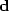data, given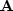generic matrix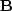generic matrixconjugate transpose of generic matrixidentity matrix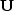unitary or pseudounitary matrix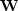weighting diagonal matrix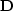diagonal matrix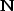NMO (normal-moveout) matrix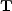tridiagonal matrix; matrix with time t on diagonal